# 具有跳跃的随机蚊子种群模型的不变测度Invariant Measure of Random Mosquito Population Model with Jumping

DOI: 10.12677/PM.2021.114075, PDF, HTML, XML, 下载: 14  浏览: 55  国家自然科学基金支持

Abstract: This paper mainly studies the invariant measures of the random mosquito population model with Markov chains. First, the Lyapunov function is cleverly constructed, and the Itô theorem and the comparison theorem are used to prove that the random mosquito population model has a unique global continuous positive solution. Second, if λ≤0, the sterile mosquito population will be extinct, and the distribution of the wild mosquito population weakly converges to the only constant probability measure; if λ > 0, then the system has an invariant probability measure, and the transition probability of the solution process converges to an invariant measure. Finally, it is proved that the transition probability of a stochastic process converges to the exponential convergence rate of its invariant measure.

1. 引言

$\left\{\begin{array}{l}\frac{\text{d}w}{\text{d}t}=\left[C\left(N\right)\frac{aw}{w+g}-\left({\mu }_{1}+{\xi }_{1}\left(w+g\right)\right)\right]w,\hfill \\ \frac{\text{d}g}{\text{d}t}=B\left(\cdot \right)-\left[{\mu }_{2}+{\xi }_{2}\left(w+g\right)\right]g.\hfill \end{array}$ (1.1)

$\left\{\begin{array}{l}\text{d}w\left(t\right)=\left[\frac{aw\left(t\right)}{1+w\left(t\right)+g\left(t\right)}-\left({\mu }_{1}+{\xi }_{1}\left(w\left(t\right)+g\left(t\right)\right)\right)\right]w\left(t\right)\text{d}t+{\sigma }_{1}w\left(t\right)\text{d}{B}_{1}\left(t\right)\hfill \\ \text{d}g\left(t\right)=\frac{bw\left(t\right)}{1+w\left(t\right)}-\left[{\mu }_{2}+{\xi }_{2}\left(w\left(t\right)+g\left(t\right)\right)\right]g\left(t\right)\text{d}t+{\sigma }_{2}g\left(t\right)\text{d}{B}_{2}\left(t\right),\hfill \end{array}$ (1.2)

$\left\{\begin{array}{l}\text{d}w\left(t\right)=\left[\frac{a\left(\alpha \left(t\right)\right)w\left(t\right)}{1+w\left(t\right)+g\left(t\right)}-{\mu }_{1}\left(w\left(t\right),\alpha \left(t\right)\right)-\left(w\left(t\right)+g\left(t\right)\right){\xi }_{1}\left(w\left(t\right),g\left(t\right),\alpha \left(t\right)\right)\right]w\left(t\right)\text{d}t\\ \text{\hspace{0.17em}}\text{\hspace{0.17em}}\text{\hspace{0.17em}}\text{\hspace{0.17em}}\text{\hspace{0.17em}}\text{\hspace{0.17em}}\text{\hspace{0.17em}}\text{\hspace{0.17em}}\text{\hspace{0.17em}}\text{\hspace{0.17em}}\text{\hspace{0.17em}}+{\sigma }_{1}\left(\alpha \left(t\right)\right)w\left(t\right)\text{d}{B}_{1}\left(t\right)\\ \text{d}g\left(t\right)=\frac{b\left(\alpha \left(t\right)\right)w\left(t\right)}{1+w\left(t\right)}-\stackrel{˜}{k}\left(\alpha \left(t\right)\right)g\left(t\right)\text{d}t+{\sigma }_{2}\left(\alpha \left(t\right)\right)g\left(t\right)\text{d}{B}_{2}\left(t\right),\end{array}$ (1.3)

$P\left\{\alpha \left(t+\Delta \right)=j|\alpha \left(t\right)=i,{X}_{t}=x\right\}=\left\{\begin{array}{l}{\gamma }_{ij}\left(x\right)\Delta +ο\left(\Delta \right),i\ne j\\ 1+{\gamma }_{ij}\left(x\right)\Delta +ο\left(\Delta \right),i=j\end{array}$ (1.4)

2. 预备知识

$\begin{array}{l}{a}^{*}=\underset{i\in \mathcal{M}}{\mathrm{max}}a\left(i\right),\text{\hspace{0.17em}}\text{\hspace{0.17em}}{b}^{*}=\underset{i\in \mathcal{M}}{\mathrm{max}}b\left(i\right),\text{\hspace{0.17em}}\text{\hspace{0.17em}}{\sigma }_{k}^{*}=\underset{i\in \mathcal{M}}{\mathrm{max}}\sigma \left(i\right),\text{\hspace{0.17em}}\text{\hspace{0.17em}}{\stackrel{˜}{k}}^{*}=\underset{i\in \mathcal{M}}{\mathrm{max}}\stackrel{˜}{k}\left(i\right),\\ {a}_{*}=\underset{i\in \mathcal{M}}{\mathrm{min}}a\left(i\right),\text{\hspace{0.17em}}\text{\hspace{0.17em}}{b}_{*}=\underset{i\in \mathcal{M}}{\mathrm{min}}b\left(i\right),\text{\hspace{0.17em}}\text{\hspace{0.17em}}{\sigma }_{k*}=\underset{i\in \mathcal{M}}{\mathrm{min}}\sigma \left(i\right),\text{\hspace{0.17em}}\text{\hspace{0.17em}}{\stackrel{˜}{k}}_{*}=\underset{i\in \mathcal{M}}{\mathrm{min}}\stackrel{˜}{k}\left(i\right).\end{array}$

${R}_{+}=\left[0,\infty \right)$${R}_{+}^{o}=\left(0,\infty \right)$${R}_{+}^{2}=\left[0,\infty \right)×\left[0,\infty \right)$${R}_{+}^{2,o}=\left(0,\infty \right)×\left(0,\infty \right)$，系统(1.3)与(1.4)的随机过程 $\left(w\left(t\right),g\left(t\right),\alpha \left(t\right)\right)$ 的相关算子：

$\mathcal{L}V\left(\varphi ,i\right)={V}_{\varphi }\left(\varphi ,i\right)\stackrel{˜}{f}\left(\varphi ,i\right)+\frac{1}{2}tr\left[\stackrel{˜}{g}\left(\varphi ,i\right){\stackrel{˜}{g}}^{\text{T}}\left(\varphi ,i\right){V}_{\varphi \varphi }\left(\varphi ,i\right)\right]+\underset{j\in M}{\sum }\text{ }\text{ }{\gamma }_{ij}V\left(\varphi ,i\right).$ (2.1)

$\stackrel{˜}{f}\left(\varphi ,i\right)={\left(\frac{a\left(i\right){w}^{2}}{1+w+g}-w{\mu }_{1}\left(w,i\right)-w\left(w+g\right){\xi }_{1}\left(w,g,i\right),\frac{wb\left(w,g,i\right)}{1+w}-g\stackrel{˜}{k}\left(i\right)\right)}^{\text{T}}$

$\stackrel{˜}{g}\left(\varphi ,i\right)=diag\left({\sigma }_{1}\left(i\right)w,{\sigma }_{2}\left(i\right)g\right)\in {R}^{2×2}$

1) ${B}_{1}\left(t\right)$${B}_{2}\left(t\right)$ 是独立于马尔科夫链 $\alpha \left(t\right)$ 的实值标准布朗运动。

2) 对任意 $i\in \mathcal{M}$$a\left(i\right),b\left(i\right),\stackrel{˜}{k}\left(i\right),{\sigma }_{1}\left(i\right),{\sigma }_{2}\left(i\right)$ 是非负的。

3) ${\mu }_{1}\left(w,i\right),{\xi }_{1}\left(w,g,i\right),b\left(w,g,i\right)$ 满足局部利普希茨条件； $\mu \left(0,i\right)={\xi }_{1}\left(0,g,i\right)=0$ 意味着对于 ${k}_{0}>1$$\underset{w\to \infty }{\mathrm{lim}}{\mu }_{1}\left(w,i\right)=\infty$$0\le b\left(w,g,i\right)\le {\kappa }_{0}\left({\mu }_{1}\left(w,i\right)\wedge {\xi }_{1}\left(w,g,i\right)\right)$${\xi }_{1}\left(w,g,i\right)\le {\kappa }_{0}\left(1+w\right)$。此外，对每个 $i\in \mathcal{M}$$b\left(w,g,i\right)$$g=0$ 时是一致连续的，即 $\underset{g\to 0}{\mathrm{lim}}\mathrm{sup}|b\left(w,g,i\right)-b\left(w,0,i\right)|=0$

4) 马尔科夫链或它的生成元 $\Gamma ={\left({\gamma }_{ij}\right)}_{N×N}$ 是不可约的，即对任意的 $i,j\in \mathcal{M}$，存在 $i={i}_{0},{i}_{1},\cdots ,{i}_{n}=j$ 使 ${\gamma }_{{i}_{k-1}},{i}_{k}>0,\text{\hspace{0.17em}}k=1,2,\cdots ,n$

1) 对于每个 $i\in \mathcal{M}$，任意的 $\left(w,g\right)\in \left[0,\infty \right)×\left(0,\infty \right)$$b\left(w,g,i\right)$ 在w上是非减的，在g上是非增的；

2) $\underset{i\in \mathcal{M}}{\sum }\left(\underset{s\to \infty }{\mathrm{lim}}\mathrm{sup}b\left(w,0,i\right)-\stackrel{˜}{k}\left(i\right)-\frac{{\sigma }_{2}^{2}\left(i\right)}{2}\right){\pi }_{\alpha }\left(i\right)<0;$ (2.2)

3) $\underset{i\in \mathcal{M}}{\sum }\left(\underset{s\to \infty }{\mathrm{lim}}\mathrm{inf}b\left(w,0,i\right)-\stackrel{˜}{k}\left(i\right)-\frac{{\sigma }_{2}^{2}\left(i\right)}{2}\right){\pi }_{\alpha }\left(i\right)>0.$ (2.3)

3. 存在性与不变测度

${P}_{w,g,i}\left\{w\left(t\right)>0,t>0\right\}=1$${P}_{w,g,i}\left\{g\left(t\right)=0,t>0\right\}=1$，如果 $g>0$，则 ${P}_{w,g,i}\left\{g\left(t\right)>0,t>0\right\}=1$

${\tau }_{e}=\mathrm{inf}\left\{t\ge 0:w\left(t\right)\vee g\left(t\right)=\infty \right\}$$\mathrm{inf}\varphi =\infty$，且解是一个强马尔科夫过程 。定义

${\tau }_{k}=\mathrm{inf}\left\{t\ge 0:w\left(t\right)\vee g\left(t\right)>k\right\},$

${\tau }_{e}={\mathrm{lim}}_{k\to \infty }{\tau }_{k}$

$\begin{array}{c}\mathcal{L}{\stackrel{˜}{V}}_{1}\left(w,g,i\right)=\frac{{\kappa }_{0}a\left(i\right){w}^{2}}{1+w+g}-{\kappa }_{0}w{\mu }_{1}\left(w,i\right)-\stackrel{˜}{k}\left(i\right)g\\ \text{\hspace{0.17em}}+w\left[\frac{b\left(w,g,i\right)}{1+w}-{\kappa }_{0}\left(w+g\right){\xi }_{1}\left(w,g,i\right)\right]\\ \le \frac{{\kappa }_{0}a\left(i\right){w}^{2}}{1+w+g}\end{array}$

${P}_{w,g,i}\left\{{\tau }_{k}

${\mu }_{1}^{\left(n\right)}\left(w,i\right)={\mu }_{1}\left(w\wedge n,i\right)$${\xi }_{1}^{\left(n\right)}\left(w,g,i\right)={\xi }_{1}\left(w\wedge n,g\wedge n,i\right)$${b}^{\left(n\right)}\left(w,g,i\right)=b\left(w\wedge n,g\wedge n,i\right)$。特别地，让

${\mu }_{1}^{\left(n\right)},{\xi }_{1}^{\left(n\right)},{b}^{\left(n\right)}$ 替代 ${\mu }_{1},{\xi }_{1},b$$\left({w}^{\left(n\right)}\left(t\right),{g}^{\left(n\right)}\left(t\right)\right)$ 成为系统(1.3)与(1.4)的解。注意

$\begin{array}{r}\hfill {\eta }^{\left(n\right)}=\mathrm{inf}\left\{t\ge 0:{w}^{\left(n\right)}\left(t\right)\wedge {g}^{\left(n\right)}\left(t\right)\le 0\right\}\end{array}$

$\begin{array}{r}\hfill {\eta }_{k}^{\left(n\right)}=\mathrm{inf}\left\{t\ge 0:{w}^{\left(n\right)}\left(t\right)\wedge {g}^{\left(n\right)}\left(t\right)<\frac{1}{k}\right\}\end{array}$

$\begin{array}{r}\hfill {\stackrel{^}{V}}_{2}^{\left(n\right)}\left(w,g,i\right)=w-{c}_{1}^{\left(n\right)}-{c}_{1}^{\left(n\right)}\mathrm{ln}\frac{w}{{c}_{1}^{\left(n\right)}}+{c}_{2}\left(g-\mathrm{ln}g-1\right),\end{array}$

$\begin{array}{l}\mathcal{L}{\stackrel{^}{V}}_{2}^{\left(n\right)}\left(w,g,i\right)\\ =\left(1-\frac{{c}_{1}^{\left(n\right)}}{g}\right)\left(\frac{a\left(i\right){w}^{2}}{1+w+g}-w{\mu }_{1}^{\left(n\right)}\left(w,i\right)-w\left(w+g\right){\xi }_{1}^{\left(n\right)}\left(w,g,i\right)\right)+\frac{{c}_{1}^{\left(n\right)}{\sigma }_{1}^{2}\left(i\right)}{2}\\ \text{\hspace{0.17em}}\text{\hspace{0.17em}}+\left({c}_{2}-\frac{{c}_{2}}{g}\right)\left(\frac{w{b}^{\left(n\right)}\left(w,g,i\right)}{1+w+g}-g\stackrel{˜}{k}\left(i\right)\right)+\frac{{c}_{2}{\sigma }_{2}^{2}\left(i\right)}{2}\end{array}$

$\begin{array}{l}\le \frac{a\left(i\right){w}^{2}}{1+w+g}+\frac{{c}_{1}^{\left(n\right)}w{\mu }_{1}^{\left(n\right)}\left(w,i\right)}{g}-w{\mu }_{1}^{\left(n\right)}\left(w,i\right)+\frac{{c}_{1}^{\left(n\right)}{\stackrel{^}{\sigma }}_{1}^{2}+{c}_{2}{\stackrel{^}{\sigma }}_{2}^{2}}{2}+{c}_{2}\stackrel{^}{k}\\ \text{\hspace{0.17em}}\text{\hspace{0.17em}}+w\left[\frac{{c}_{1}^{\left(n\right)}\left(w+g\right){\xi }_{1}^{\left(n\right)}\left(w,g,i\right)}{g}-\frac{{c}_{2}{b}^{\left(n\right)}\left(w,g,i\right)}{g\left(1+w\right)}\right]\\ \text{\hspace{0.17em}}\text{\hspace{0.17em}}+w\left[\frac{{c}_{2}{b}^{\left(n\right)}\left(w,g,i\right)}{1+w}-\left(w+g\right){\xi }_{1}^{\left(n\right)}\left(w,g,i\right)\right]\\ \le \frac{a\left(i\right){w}^{2}}{1+w+g}+\frac{{c}_{1}^{\left(n\right)}{c}_{2}^{\left(n\right)}}{g}-{c}_{2}^{\left(2\right)}+\frac{{c}_{1}^{\left(n\right)}{\stackrel{^}{\sigma }}_{1}^{2}+{c}_{2}{\stackrel{^}{\sigma }}_{2}^{2}}{2}:={K}^{\left(n\right)};\text{\hspace{0.17em}}\text{\hspace{0.17em}}w,g>0,\end{array}$

$\begin{array}{l}{E}_{w,g,i}{\stackrel{^}{V}}_{2}^{\left(n\right)}\left({w}^{\left(n\right)}\left({\eta }_{k}^{\left(n\right)}\wedge t\right),{g}^{\left(n\right)}\left({\eta }_{k}^{\left(n\right)}\wedge t\right),{\alpha }^{\left(n\right)}\left({\eta }_{k}^{\left(n\right)}\wedge t\right)\right)\\ ={\stackrel{^}{V}}_{2}^{\left(n\right)}\left(w,g,i\right)+{E}_{w,g,i}{\int }_{0}^{{\eta }_{k}^{\left(n\right)}\wedge t}\mathcal{L}{\stackrel{^}{V}}_{2}^{\left(n\right)}\left({w}^{\left(n\right)}\left(u\right),{g}^{\left(n\right)}\left(u\right),{\alpha }^{\left(n\right)}\left(u\right)\right)\text{d}u\\ \le {\stackrel{^}{V}}_{2}^{\left(n\right)}\left(w,g,i\right)+{K}^{\left(n\right)}t.\end{array}$

${\stackrel{^}{V}}_{2}^{\left(n\right)}\left({w}^{\left(n\right)}\left({\eta }_{k}^{\left(n\right)}\wedge t\right),{g}^{\left(n\right)}\left({\eta }_{k}^{\left(n\right)}\wedge t\right),{\alpha }^{\left(n\right)}\left({\eta }_{k}^{\left(n\right)}\wedge t\right)\right)\ge \left({c}_{1}^{\left(n\right)}\mathrm{ln}k{c}_{1}^{\left(n\right)}-{c}_{1}^{\left(n\right)}\right)\wedge \left({c}_{2}\mathrm{ln}k-{c}_{2}\right).$

${P}_{w,g,i}\left\{{\eta }_{k}^{\left(n\right)}

$\begin{array}{r}\hfill {P}_{w,g,i}\left\{{w}^{\left(n\right)}\left(t\right),{g}^{\left(n\right)}\left(t\right)>0:\forall t>0\right\}=1.\end{array}$

$w\left(y\right)\left(\omega \right)\vee g\left(y\right)\left(\omega \right)<{n}_{0};\text{\hspace{0.17em}}\text{\hspace{0.17em}}\forall 0\le y\le t.$

$\begin{array}{l}w\left(t\right)\left(\omega \right)={w}^{\left({n}_{0}\right)}\left(t\right)\left(\omega \right)>0,\\ g\left(t\right)\left(\omega \right)={g}^{\left({n}_{0}\right)}\left(t\right)\left(\omega \right)>0.\end{array}$

${P}_{w,g,i}\left\{w\left(t\right)>0:t>0\right\}={P}_{w,g,i}\left\{g\left(t\right)>0:t>0\right\}=1;\forall w,g>0.$ (3.1)

$\frac{a\left(i\right){\stackrel{˜}{w}}^{2}}{1+\stackrel{˜}{w}+g}-\stackrel{˜}{w}{\mu }_{1}\left(\stackrel{˜}{w},i\right)-\stackrel{˜}{w}\left(\stackrel{˜}{w}+g\right){\xi }_{1}\left(\stackrel{˜}{w},g,i\right)\ge 0.$ (3.2)

${\stackrel{˜}{\tau }}_{1}=\mathrm{inf}\left\{t>0:w\left(t\right)+|g\left(t\right)-g|\ge \epsilon \right\}.$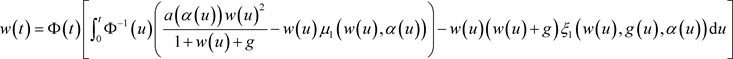(3.3)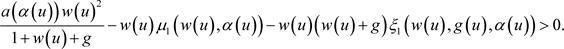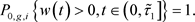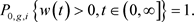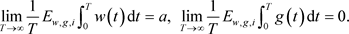(3.4)

i) 对于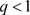的情况，对任意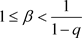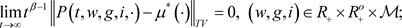(3.5)

ii) 对于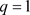的情况，此时存在一个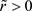，有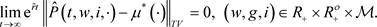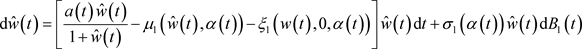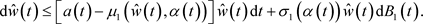(3.6)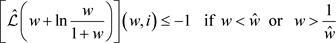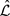是方程(3.6)的相关算子。由于它的非退化性，方程(3.6)的扩散系数是正常返的，且系统(3.6)存在唯一不变测度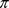。此外，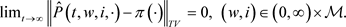(3.7)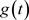很小时，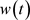可以用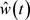近似表示。由伊藤公式和随机过程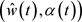的遍历性，当很小时，它的长期增长率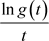可以由临界值近似得到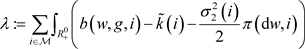(3.8)

1)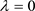的情况。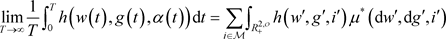(3.9)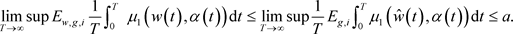(3.10)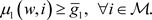(3.11)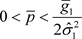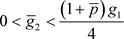通过伊藤公式，得到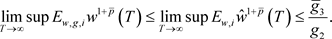(3.12)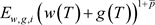是在T中一致有界的. (3.13)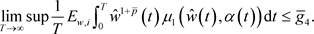(3.14)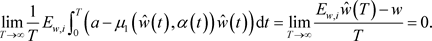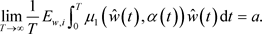(3.15)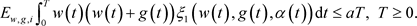(3.16)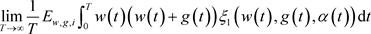(3.17)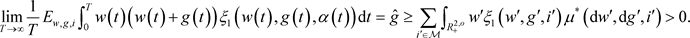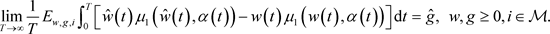(3.18)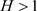为一个充分大的常数并且满足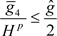，得到(3.19)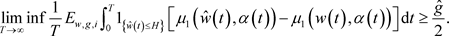(3.20)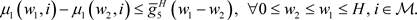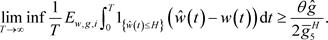(3.21)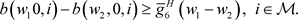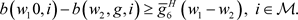(3.22)(3.23)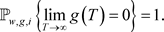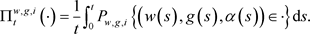2）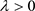的情况。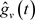成为以下方程的解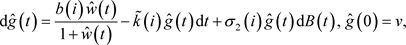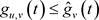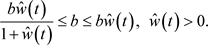3)的情况。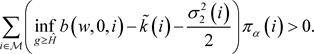(3.24)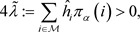(3.25)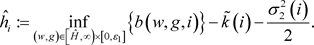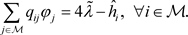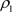满足充分小，有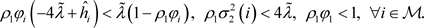(3.26)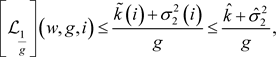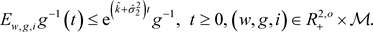(3.27)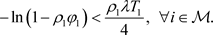(3.28)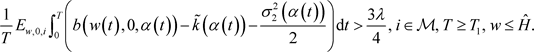(3.29)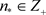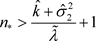，根据( ，命题4.1)，存在一些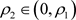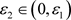，满足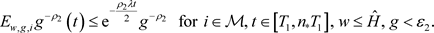(3.30)(3.31)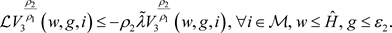(3.32)(3.33)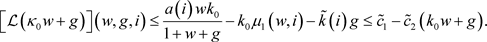(3.34)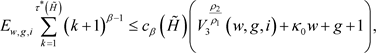(3.35)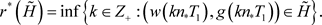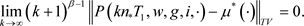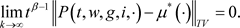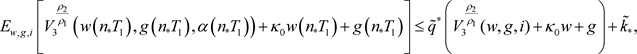，有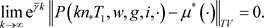Benelli, G. and Mehlhorn, H. (2018) Mosquito Borne Diseases Implications for Public Health. Springer, Berlin. https://doi.org/10.1007/978-3-319-94075-5  Fernandes, J., Moise, I., et al. (2018) Revamping Mosquito-Borne Disease Control to Tackle Future Threats. Trends in Parasitology, 34, 359-368. https://doi.org/10.1016/j.pt.2018.01.005  Benelli, G., Caselli, A. and Canale, A. (2017) Nanoparticles for Mosquito Control: Challenges and Constraints. Journal of King Saud University—Science, 29, 424-435. https://doi.org/10.1016/j.jksus.2016.08.006  Blayneh, K. and Mohammed-Awel, J. (2014) Insecticide-Resistant Mosquitoes and Malaria Control. Mathematical Biosciences, 25, 14-26. https://doi.org/10.1016/j.mbs.2014.03.007  Iturbe-Ormaetxe, I., et al. (2011) Wolbachia and the Biological Control of Mosquito-Borne Disease. EMBO Reports, 12, 508-518. https://doi.org/10.1038/embor.2011.84  Alphey, L. (2014) Genetic Control of Mosquitoes. Annual Review of Entomology, 59, 205-224. https://doi.org/10.1146/annurev-ento-011613-162002  Wilke, A., Bruno, B., et al. (2012) Genetic Control of Mosquitoes: Population Suppression Strategies. Revista do Instituto de Medicina Tropical de Sao Paulo, 54, 287-292. https://doi.org/10.1590/S0036-46652012000500009  McLean, K. and Jacobs-Lorena, M. (2016) Genetic Control of Malaria Mosquitoes. Trends in Parasitology, 32, 174-176. https://doi.org/10.1016/j.pt.2016.01.002  Cai, L., Ai, S. and Li, J. (2014) Dynamics of Mosquitoes Populations with Different Strategies for Releasing Sterile Mosquitoes. SIAM Journal on Applied Mathematics, 74, 1786-1809. https://doi.org/10.1137/13094102X  Scheuring, I. (1999) Allee Effect Increases the Dynamical Stability of Populations. Journal of Theoretical Biology, 198, 407-414. https://doi.org/10.1006/jtbi.1999.0966  Zhu, C. and Yin, G. (2009) On Strong Feller, Recurrence, and Weak Stabilization of Regime-Switching Diffusions. SIAM Journal on Control and Optimization, 48, 2003-2031. https://doi.org/10.1137/080712532  Kabouris, J. and Georgakakos, A. (1996) Parameter and State Estimation of the Activated Sludge Process I. Model Development. Water Research, 30, 2853-2865. https://doi.org/10.1016/0043-1354(95)00325-8  Hening, A. and Nguyen, D. (2018) Coexistence and Extinction for Stochastic Kolmogorov Systems. Annals of Applied Probability, 28, 1893-1942. https://doi.org/10.1214/17-AAP1347  Fort, G. and Roberts, G. (2005) Subgeometric Ergodicity of Strong Markov Processes. Annals of Applied Probability, 15, 1565-1589. https://doi.org/10.1214/105051605000000115  Benaim, M. (2018) Stochastic Persistence.  Tuominen, P. and Tweedie, R. (1994) Subgeometric Rates of Convergence of f-Ergodic Markov Chains. Advances in Applied Prob-ability, 26, 775-798. https://doi.org/10.2307/1427820